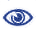# Prague Stringology Conference 2016

Dynamic Index and LZ Factorization in Compressed Space

Abstract:
In this paper, we propose a new dynamic compressed index of O(w) space for a dynamic text T, where w = O(min(z log N log* M, N)) is the size of the signature encoding of T, z is the size of the Lempel-Ziv77 (LZ77) factorization of T, N is the length of T, and M ≥ 4N is an integer that can be handled in constant time under word RAM model. Our index supports searching for a pattern P in T in O(|P| f + log w log |P| log* M (log N + log |P| log* M) + occ log N) time and insertion/deletion of a substring of length y in O((y + log N log* M) log w log N log* M) time, where f = O(min {
 log log M log log w log log log M
,
 log w log log w
}). Also, we propose a new space-efficient LZ77 factorization algorithm for a given text of length N, which runs in O(N f + z log w log3 N (log* N)2) time with O(w) working space.

 Download paper:PostScript PDF BibTeX reference
 Download presentation: